UPDATE: I did a talk on property-based testing based on these posts. Slides and video here.

In the previous post, I described the basics of property-based testing, and showed how it could save a lot of time by generating random tests.

But here’s a common problem. Everyone who sees a property-based testing tool like FsCheck or QuickCheck thinks that it is amazing… but when it times come to start creating your own properties, the universal complaint is: “what properties should I use? I can’t think of any!”

The goal of this post is to show some common patterns that can help you discover the properties that are applicable to your code.

## Categories for properties

In my experience, many properties can be discovered by using one of the seven approaches listed below.

This is by no means a comprehensive list, just the ones that have been most useful to me. For a different perspective, check out the list of patterns that the PEX team at Microsoft have compiled.

### “Different paths, same destination”

These kinds of properties are based on combining operations in different orders, but getting the same result. For example, in the diagram below, doing `X` then `Y` gives the same result as doing `Y` followed by `X`.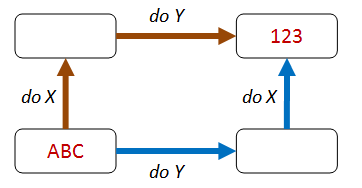The commutative property of addition is an obvious example of this pattern. For example, the result of `add 1` then `add 2` is the same as the result of `add 2` followed by `add 1`.

This pattern, generalized, can produce a wide range of useful properties. We’ll see some more uses of this pattern later in this post.

### “There and back again”

These kinds of properties are based on combining an operation with its inverse, ending up with the same value you started with.

In the diagram below, doing `X` serializes `ABC` to some kind of binary format, and the inverse of `X` is some sort of deserialization that returns the same `ABC` value again.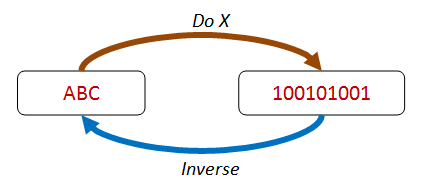In addition to serialization/deserialization, other pairs of operations can be checked this way: `addition`/`subtraction`, `write`/`read`, `setProperty`/`getProperty`, and so on.

Other pair of functions fit this pattern too, even though they are not strict inverses, pairs such as `insert`/`contains`, `create`/`exists` , etc.

### “Some things never change”

These kinds of properties are based on an invariant that is preserved after some transformation.

In the diagram below, the transform changes the order of the items, but the same four items are still present afterwards.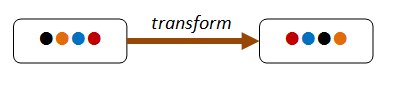Common invariants include size of a collection (for `map` say), the contents of a collection (for `sort` say), the height or depth of something in proportion to size (e.g. balanced trees).

### “The more things change, the more they stay the same”

These kinds of properties are based on “idempotence” – that is, doing an operation twice is the same as doing it once.

In the diagram below, using `distinct` to filter the set returns two items, but doing `distinct` twice returns the same set again.Idempotence properties are very useful, and can be extended to things like database updates and message processing.

### “Solve a smaller problem first”

These kinds of properties are based on “structural induction” – that is, if a large thing can be broken into smaller parts, and some property is true for these smaller parts, then you can often prove that the property is true for a large thing as well.

In the diagram below, we can see that the four-item list can be partitioned into an item plus a three-item list, which in turn can be partitioned into an item plus a two-item list. If we can prove the property holds for two-item list, then we can infer that it holds for the three-item list, and for the four-item list as well.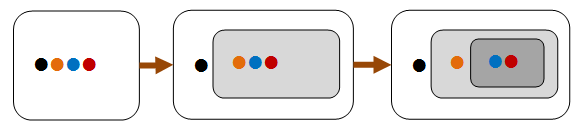Induction properties are often naturally applicable to recursive structures such as lists and trees.

### “Hard to prove, easy to verify”

Often an algorithm to find a result can be complicated, but verifying the answer is easy.

In the diagram below, we can see that finding a route through a maze is hard, but checking that it works is trivial!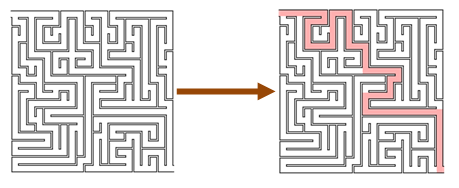Many famous problems are of this sort, such as prime number factorization. But this approach can be used for even simple problems.

For example, you might check that a string tokenizer works by just concatenating all the tokens again. The resulting string should be the same as what you started with.

### “The test oracle”

In many situations you often have an alternate version of an algorithm or process (a “test oracle”) that you can use to check your results.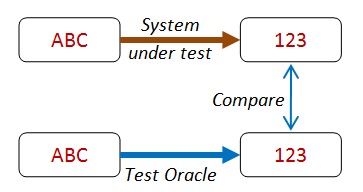For example, you might have a high-performance algorithm with optimization tweaks that you want to test. In this case, you might compare it with a brute force algorithm that is much slower but is also much easier to write correctly.

Similarly, you might compare the result of a parallel or concurrent algorithm with the result of a linear, single thread version.

## Putting the categories to work with some real examples

In this section, we’ll apply these categories to see if we can come up with properties for some simple functions such as “sort a list” and “reverse a list”.

### “Different paths, same destination” applied to a list sort

Let’s start with “different paths, same destination” and apply it to a “list sort” function.

Can we think of any way of combining an operation before `List.sort`, and another operation after `List.sort`, so that you should end up with the same result? That is, so that “going up then across the top” is the same as “going across the bottom then up”.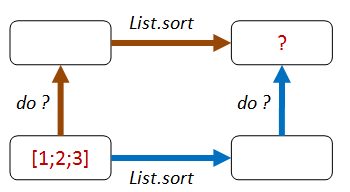• Path 1: We add one to each element of the list, then sort.
• Path 2: We sort, then add one to each element of the list.
• Both lists should be equal.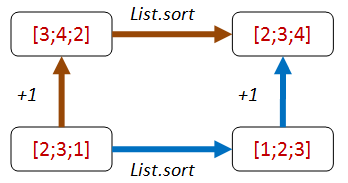Here’s some code that implements that property:

``````let ``+1 then sort should be same as sort then +1`` sortFn aList =
let add1 x = x + 1

let result1 = aList |> sortFn |> List.map add1
let result2 = aList |> List.map add1 |> sortFn
result1 = result2

// test
let goodSort = List.sort
Check.Quick (``+1 then sort should be same as sort then +1`` goodSort)
// Ok, passed 100 tests.
``````

Well, that works, but it also would work for a lot of other transformations too. For example, if we implemented `List.sort` as just the identity, then this property would be satisfied equally well! You can test this for yourself:

``````let badSort aList = aList
Check.Quick (``+1 then sort should be same as sort then +1`` badSort)
// Ok, passed 100 tests.
``````

The problem with this property is that it is not exploiting any of the “sortedness”. We know that a sort will probably reorder a list, and certainly, the smallest element should be first.

How about adding an item that we know will come at the front of the list after sorting?

• Path 1: We append `Int32.MinValue` to the end of the list, then sort.
• Path 2: We sort, then prepend `Int32.MinValue` to the front of the list.
• Both lists should be equal.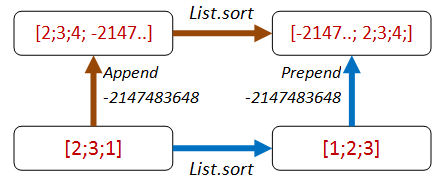Here’s the code:

``````let ``append minValue then sort should be same as sort then prepend minValue`` sortFn aList =
let minValue = Int32.MinValue

let appendThenSort = (aList @ [minValue]) |> sortFn
let sortThenPrepend = minValue :: (aList |> sortFn)
appendThenSort = sortThenPrepend

// test
Check.Quick (``append minValue then sort should be same as sort then prepend minValue`` goodSort)
// Ok, passed 100 tests.
``````

``````Check.Quick (``append minValue then sort should be same as sort then prepend minValue`` badSort)
// Falsifiable, after 1 test (2 shrinks)
// 
``````

In other words, the bad sort of `[0; minValue]` is not the same as `[minValue; 0]`.

So that’s good!

But… we’ve got some hard coded things in there that the Enterprise Developer From Hell (see previous post) could take advantage of! The EDFH will exploit the fact that we always use `Int32.MinValue` and that we always prepend or append it to the test list.

In other words, the EDFH can identify which path we are on and have special cases for each one:

``````// The Enterprise Developer From Hell strikes again
match aList with
| [] -> []
| _ ->
let last::reversedTail = List.rev aList
if (last = Int32.MinValue) then
// if min is last, move to front
let unreversedTail = List.rev reversedTail
last :: unreversedTail
else
aList // leave alone
``````

And when we check it…

``````// Oh dear, the bad implementation passes!
Check.Quick (``append minValue then sort should be same as sort then prepend minValue`` badSort2)
// Ok, passed 100 tests.
``````

We could fix this by (a) picking a random number smaller than any number in the list and (b) inserting it at a random location rather than always appending it. But rather than getting too complicated, let’s stop and reconsider.

An alternative approach which also exploits the “sortedness” is to first negate all the values, then on the path that negates after the sort, add an extra reverse as well.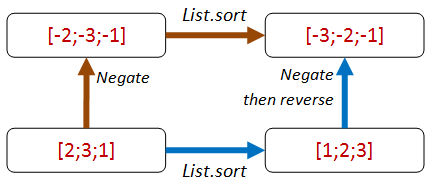``````let ``negate then sort should be same as sort then negate then reverse`` sortFn aList =
let negate x = x * -1

let negateThenSort = aList |> List.map negate |> sortFn
let sortThenNegateAndReverse = aList |> sortFn |> List.map negate |> List.rev
negateThenSort = sortThenNegateAndReverse
``````

This property is harder for the EDFH to beat because there are no magic numbers to help identify which path you are on:

``````// test
Check.Quick ( ``negate then sort should be same as sort then negate then reverse`` goodSort)
// Ok, passed 100 tests.

// test
Check.Quick ( ``negate then sort should be same as sort then negate then reverse``  badSort)
// Falsifiable, after 1 test (1 shrinks)
// [1; 0]

// test
Check.Quick ( ``negate then sort should be same as sort then negate then reverse``  badSort2)
// Falsifiable, after 5 tests (3 shrinks)
// [1; 0]
``````

You might argue that we are only testing sorting for lists of integers. But the `List.sort` function is generic and knows nothing about integers per se, so I have high confidence that this property does test the core sorting logic.

### Applying “different paths, same destination” to a list reversal function

Ok, enough of `List.sort`. What about applying the same ideas to the list reversal function?

We can do the same append/prepend trick: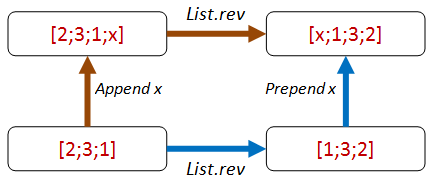Here’s the code for the property:

``````let ``append any value then reverse should be same as reverse then prepend same value`` revFn anyValue aList =

let appendThenReverse = (aList @ [anyValue]) |> revFn
let reverseThenPrepend = anyValue :: (aList |> revFn)
appendThenReverse = reverseThenPrepend
``````

Here are the test results for the correct function and for two incorrect functions:

``````// test
let goodReverse = List.rev
Check.Quick (``append any value then reverse should be same as reverse then prepend same value`` goodReverse)
// Ok, passed 100 tests.

Check.Quick (``append any value then reverse should be same as reverse then prepend same value`` badReverse)
// Falsifiable, after 1 test (2 shrinks)
// true, []

Check.Quick (``append any value then reverse should be same as reverse then prepend same value`` badReverse2)
// Falsifiable, after 1 test (1 shrinks)
// true, [false]
``````

You might notice something interesting here. I never specified the type of the list. The property works with any list.

In cases like these, FsCheck will generate random lists of bools, strings, ints, etc.

In both failing cases, the `anyValue` is a bool. So FsCheck is using lists of bools to start with.

Here’s an exercise for you: Is this property good enough? Is there some way that the EDFH can create an implementation that will pass?

## “There and back again”

Sometimes the multi-path style properties are not available or too complicated, so let’s look at some other approaches.

Let’s start with list sorting again. Is there an inverse to sorting? Hmmm, not really. So we’ll skip sorting for now.

What about list reversal? Well, as it happens, reversal is its own inverse!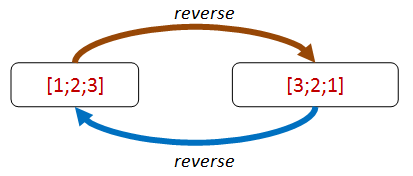Let’s turn that into a property:

``````let ``reverse then reverse should be same as original`` revFn aList =
let reverseThenReverse = aList |> revFn |> revFn
reverseThenReverse = aList
``````

And it passes:

``````let goodReverse = List.rev
Check.Quick (``reverse then reverse should be same as original`` goodReverse)
// Ok, passed 100 tests.
``````

Unfortunately, a bad implementation satisfies the property too!

``````let badReverse aList = aList
Check.Quick (``reverse then reverse should be same as original`` badReverse)
// Ok, passed 100 tests.
``````

Nevertheless, the use of properties involving inverses can be very useful to verify that your inverse function (such as deserialization) does indeed “undo” the primary function (such as serialization).

We’ll see some real examples of using this in the next post.

## “Hard to prove, easy to verify”

So far we’ve been testing properties without actually caring about the end result of an operation.

But of course in practice, we do care about the end result!

Now we normally can’t really tell if the result is right without duplicating the function under test. But often we can tell that the result is wrong quite easily. In the maze diagram from above, we can easily check whether the path works or not.

If we are looking for the shortest path, we might not be able to check it, but at least we know that we have some valid path.

This principle can be applied quite generally.

For example, let’s say that we want to check whether a `string split` function is working. We don’t have to write a tokenizer – all we have to do is ensure that the tokens, when concatenated, give us back the original string!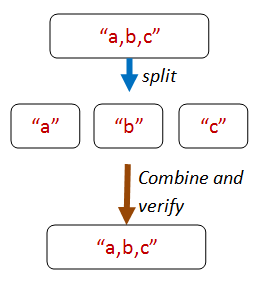Here’s the core code from that property:

``````let concatWithComma s t = s + "," + t

let tokens = originalString.Split [| ',' |]
let recombinedString =
// can use reduce safely because there is always at least one token
tokens |> Array.reduce concatWithComma

// compare the result with the original
originalString = recombinedString
``````

But how can we create an original string? The random strings generated by FsCheck are unlikely to contain many commas!

There are ways that you can control exactly how FsCheck generates random data, which we’ll look at later.

For now though, we’ll use a trick. The trick is to let FsCheck generate a list of random strings, and then we’ll build an `originalString` from them by concatting them together.

So here’s the complete code for the property:

``````let ``concatting the elements of a string split by commas recreates the original string`` aListOfStrings =
// helper to make a string
let addWithComma s t = s + "," + t
let originalString = aListOfStrings |> List.fold addWithComma ""

// now for the property
let tokens = originalString.Split [| ',' |]
let recombinedString =
// can use reduce safely because there is always at least one token

// compare the result with the original
originalString = recombinedString
``````

When we test this we are happy:

``````Check.Quick ``concatting the elements of a string split by commas recreates the original string``
// Ok, passed 100 tests.
``````

### “Hard to prove, easy to verify” for list sorting

So how can we apply this principle to a sorted list? What property is easy to verify?

The first thing that pops into my mind is that for each pair of elements in the list, the first one will be smaller than the second.So let’s make that into a property:

``````let ``adjacent pairs from a list should be ordered`` sortFn aList =
let pairs = aList |> sortFn |> Seq.pairwise
pairs |> Seq.forall (fun (x,y) -> x <= y )
``````

But something funny happens when we try to check it. We get an error!

``````let goodSort = List.sort
Check.Quick (``adjacent pairs from a list should be ordered`` goodSort)
``````
``````System.Exception: Geneflect: type not handled System.IComparable
at FsCheck.ReflectArbitrary.reflectObj@102-4.Invoke(String message)
at Microsoft.FSharp.Core.PrintfImpl.go@523-3[b,c,d](String fmt, Int32 len, FSharpFunc`2 outputChar, FSharpFunc`2 outa, b os, FSharpFunc`2 finalize, FSharpList`1 args, Int32 i)
at Microsoft.FSharp.Core.PrintfImpl.run@521[b,c,d](FSharpFunc`2 initialize, String fmt, Int32 len, FSharpList`1 args)
``````

What does `System.Exception: type not handled System.IComparable` mean? It means that FsCheck is trying to generate a random list, but all it knows is that the elements must be `IComparable`. But `IComparable` is not a type than can be instantiated, so FsCheck throws an error.

How can we prevent this from happening? The solution is to specify a particular type for the property, such as `int list`, like this:

``````let ``adjacent pairs from a list should be ordered`` sortFn (aList:int list) =
let pairs = aList |> sortFn |> Seq.pairwise
pairs |> Seq.forall (fun (x,y) -> x <= y )
``````

This code works now.

``````let goodSort = List.sort
Check.Quick (``adjacent pairs from a list should be ordered`` goodSort)
// Ok, passed 100 tests.
``````

Note that even though the property has been constrained, the property is still a very general one. We could have used `string list` instead, for example, and it would work just the same.

``````let ``adjacent pairs from a string list should be ordered`` sortFn (aList:string list) =
let pairs = aList |> sortFn |> Seq.pairwise
pairs |> Seq.forall (fun (x,y) -> x <= y )

Check.Quick (``adjacent pairs from a string list should be ordered`` goodSort)
// Ok, passed 100 tests.
``````

TIP: If FsCheck throws “type not handled”, add explicit type constraints to your property

Are we done now? No! One problem with this property is that it doesn’t catch malicious implementations by the EDFH.

``````// bad implementation passes
// Ok, passed 100 tests.
``````

Is it a surprise to you that a silly implementation also works?

Hmmm. That tells us that there must be some property other than pairwise ordering associated with sorting that we’ve overlooked. What are we missing here?

This is a good example of how doing property-based testing can lead to insights about design. We thought we knew what sorting meant, but we’re being forced to be a bit stricter in our definition.

As it happens, we’ll fix this particular problem by using the next principle!

## “Some things never change”

A useful kind of property is based on an invariant that is preserved after some transformation, such as preserving length or contents.

They are not normally sufficient in themselves to ensure a correct implementation, but they do often act as a counter-check to more general properties.

For example, in the previous post, we created commutative and associative properties for addition, but then noticed that simply having an implementation that returned zero would satisfy them just as well! It was only when we added `x + 0 = x` as a property that we could eliminate that particular malicious implementation.

And in the “list sort” example above, we could satisfy the “pairwise ordered” property with a function that just returned an empty list! How could we fix that?

Our first attempt might be to check the length of the sorted list. If the lengths are different, then the sort function obviously cheated!

``````let ``sort should have same length as original`` sortFn (aList:int list) =
let sorted = aList |> sortFn
List.length sorted = List.length aList
``````

We check it and it works:

``````let goodSort = List.sort
Check.Quick (``sort should have same length as original`` goodSort )
// Ok, passed 100 tests.
``````

And yes, the bad implementation fails:

``````let badSort aList = []
Check.Quick (``sort should have same length as original`` badSort )
// Falsifiable, after 1 test (1 shrink)
// 
``````

Unfortunately, the BDFH is not defeated and can come up with another compliant implementation! Just repeat the first element N times!

``````// bad implementation has same length
match aList with
| [] -> []

// for example
``````

Now when we test this, it passes:

``````Check.Quick (``sort should have same length as original`` badSort2)
// Ok, passed 100 tests.
``````

What’s more, it also satisfies the pairwise property too!

``````Check.Quick (``adjacent pairs from a list should be ordered`` badSort2)
// Ok, passed 100 tests.
``````

### Sort invariant - 2nd attempt

So now we have to try again. What is the difference between the real result `[1;2;3]` and the fake result `[1;1;1]`?

Answer: the fake result is throwing away data. The real result always contains the same contents as the original list, but just in a different order.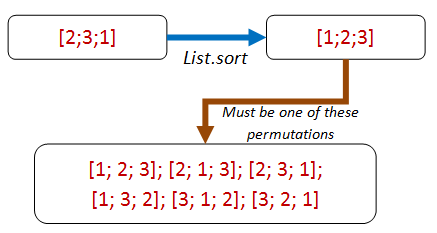That leads us to a new property: a sorted list is always a permutation of the original list. Aha! Let’s write the property in terms of permutations now:

``````let ``a sorted list is always a permutation of the original list`` sortFn (aList:int list) =
let sorted = aList |> sortFn
let permutationsOfOriginalList = permutations aList

// the sorted list must be in the seq of permutations
permutationsOfOriginalList
|> Seq.exists (fun permutation -> permutation = sorted)
``````

Great, now all we need is a permutation function.

Let’s head over to StackOverflow and steal borrow an implementation. Here it is:

``````/// given aList and anElement to insert,
/// generate all possible lists with anElement
/// inserted into aList
let rec insertElement anElement aList =
// From http://stackoverflow.com/a/4610704/1136133
seq {
match aList with
// empty returns a singleton
| [] -> yield [anElement]
// not empty?
| first::rest ->
// return anElement prepended to the list
yield anElement::aList
// also return first prepended to all the sublists
for sublist in insertElement anElement rest do
yield first::sublist
}

/// Given a list, return all permutations of it
let rec permutations aList =
seq {
match aList with
| [] -> yield []
| first::rest ->
// for each sub-permutation,
// return the first inserted into it somewhere
for sublist in permutations rest do
yield! insertElement first sublist
}
``````

Some quick interactive tests confirm that it works as expected:

``````permutations ['a';'b';'c'] |> Seq.toList
//  [['a'; 'b'; 'c']; ['b'; 'a'; 'c']; ['b'; 'c'; 'a']; ['a'; 'c'; 'b'];
//  ['c'; 'a'; 'b']; ['c'; 'b'; 'a']]

permutations ['a';'b';'c';'d'] |> Seq.toList
//  [['a'; 'b'; 'c'; 'd']; ['b'; 'a'; 'c'; 'd']; ['b'; 'c'; 'a'; 'd'];
//   ['b'; 'c'; 'd'; 'a']; ['a'; 'c'; 'b'; 'd']; ['c'; 'a'; 'b'; 'd'];
//   ['c'; 'b'; 'a'; 'd']; ['c'; 'b'; 'd'; 'a']; ['a'; 'c'; 'd'; 'b'];
//   ['c'; 'a'; 'd'; 'b']; ['c'; 'd'; 'a'; 'b']; ['c'; 'd'; 'b'; 'a'];
//   ['a'; 'b'; 'd'; 'c']; ['b'; 'a'; 'd'; 'c']; ['b'; 'd'; 'a'; 'c'];
//   ['b'; 'd'; 'c'; 'a']; ['a'; 'd'; 'b'; 'c']; ['d'; 'a'; 'b'; 'c'];
//   ['d'; 'b'; 'a'; 'c']; ['d'; 'b'; 'c'; 'a']; ['a'; 'd'; 'c'; 'b'];
//   ['d'; 'a'; 'c'; 'b']; ['d'; 'c'; 'a'; 'b']; ['d'; 'c'; 'b'; 'a']]

permutations [3;3] |> Seq.toList
//  [[3; 3]; [3; 3]]
``````

Excellent! Now let’s run FsCheck:

``````Check.Quick (``a sorted list is always a permutation of the original list`` goodSort)
``````

Hmmm. That’s funny, nothing seems to be happening. And my CPU is maxing out for some reason. What’s going on?

What’s going on is that you are going to be sitting there for a long time! If you are following along at home, I suggest you right-click and cancel the interactive session now.

The innocent looking `permutations` is really really slow for any normal sized list. For example, a list of just 10 items has 3,628,800 permutations. While with 20 items, you are getting to astronomical numbers.

And of course, FsCheck will be doing hundreds of these tests! So this leads to an important tip:

TIP: Make sure your property checks are very fast. You will be running them a LOT!

We’ve already seen that even in the best case, FsCheck will evaluate the property 100 times. And if shrinking is needed, even more. So make sure your tests are fast to run!

But what happens if you are dealing with real systems such as databases, networks, or other slow dependencies?

In his (highly recommended) video on using QuickCheck, John Hughes tells of when his team was trying to detect flaws in a distributed data store that could be caused by network partitions and node failures.

Of course, killing real nodes thousands of times was too slow, so they extracted the core logic into a virtual model, and tested that instead. As a result, the code was later refactored to make this kind of testing easier. In other words, property-based testing influenced the design of the code, just as TDD would.

### Sort invariant - 3rd attempt

Ok, so we can’t use permutations by just looping through them. So let’s use the same idea but write a function that is specific for this case, a `isPermutationOf` function.

``````let ``a sorted list has same contents as the original list`` sortFn (aList:int list) =
let sorted = aList |> sortFn
isPermutationOf aList sorted
``````

Here’s the code for `isPermutationOf` and its associated helper functions:

``````/// Given an element and a list, and other elements previously skipped,
/// return a new list without the specified element.
let rec withoutElementRec anElement aList skipped =
match aList with
| [] -> None
// matched, so create a new list from the skipped and the remaining
// and return it
let skipped' = List.rev skipped
Some (skipped' @ tail)
// no match, so prepend head to the skipped and recurse
let skipped' = head :: skipped
withoutElementRec anElement tail skipped'

/// Given an element and a list
/// return a new list without the specified element.
let withoutElement x aList =
withoutElementRec x aList []

/// Given two lists, return true if they have the same contents
/// regardless of order
let rec isPermutationOf list1 list2 =
match list1 with
| [] -> List.isEmpty list2 // if both empty, true
| h1::t1 ->
match withoutElement h1 list2 with
| None -> false
| Some t2 ->
isPermutationOf t1 t2
``````

Let’s try the test again. And yes, this time it completes before the heat death of the universe.

``````Check.Quick (``a sorted list has same contents as the original list``  goodSort)
// Ok, passed 100 tests.
``````

What’s also great is that the malicious implementation now fails to satisfy this property!

``````Check.Quick (``a sorted list has same contents as the original list``  badSort2)
// Falsifiable, after 2 tests (5 shrinks)
// [1; 0]
``````

In fact, these two properties, `adjacent pairs from a list should be ordered` and `a sorted list has same contents as the original list` should indeed ensure that any implementation is correct.

Just above, we noted that there were two properties needed to define the “is sorted” property. It would be nice if we could combine them into one property `is sorted` so that we can have a single test.

Well, of course we can always merge the two sets of code into one function, but it’s preferable to keep functions as small as possible. Furthermore, a property like `has same contents` might be reusable in other contexts as well.

What we want then, is an equivalent to `AND` and `OR` that is designed to work with properties.

FsCheck to the rescue! There are built in operators to combine properties: `.&.` for `AND` and `.|.` for `OR`.

Here is an example of them in use:

``````let ``list is sorted``sortFn (aList:int list) =
let prop1 = ``adjacent pairs from a list should be ordered`` sortFn aList
let prop2 = ``a sorted list has same contents as the original list`` sortFn aList
prop1 .&. prop2
``````

When we test the combined property with a good implementation of `sort`, everything works as expected.

``````let goodSort = List.sort
Check.Quick (``list is sorted`` goodSort )
// Ok, passed 100 tests.
``````

And if we test a bad implementation, the combined property fails as well.

``````let badSort aList = []
Check.Quick (``list is sorted`` badSort )
// Falsifiable, after 1 test (0 shrinks)
// 
``````

But there’s a problem now. Which of the two properties failed?

What we would like to do is add a “label” to each property so that we can tell them apart. In FsCheck, this is done with the `|@` operator:

``````let ``list is sorted (labelled)``sortFn (aList:int list) =
let prop1 = ``adjacent pairs from a list should be ordered`` sortFn aList
|@ "adjacent pairs from a list should be ordered"
let prop2 = ``a sorted list has same contents as the original list`` sortFn aList
|@ "a sorted list has same contents as the original list"
prop1 .&. prop2
``````

And now, when we test with the bad sort, we get a message `Label of failing property: a sorted list has same contents as the original list`:

``````Check.Quick (``list is sorted (labelled)`` badSort )
//  Falsifiable, after 1 test (2 shrinks)
//  Label of failing property: a sorted list has same contents as the original list
//  
``````

For more on these operators, see the FsCheck documentation under “And, Or and Labels”.

And now, back to the property-divising strategies.

## “Solving a smaller problem”

Sometimes you have a recursive data structure or a recursive problem. In these cases, you can often find a property that is true of a smaller part.

For example, for a sort, we could say something like:

``````A list is sorted if:
* The first element is smaller (or equal to) the second.
* The rest of the elements after the first element are also sorted.
``````

Here is that logic expressed in code:

``````let rec ``First element is <= than second, and tail is also sorted`` sortFn (aList:int list) =
let sortedList = aList |> sortFn
match sortedList with
| [] -> true
| [first] -> true
| [first;second] ->
first <= second
| first::second::tail ->
first <= second &&
let subList = second::tail
``First element is <= than second, and tail is also sorted`` sortFn subList
``````

This property is satisfied by the real sort function:

``````let goodSort = List.sort
Check.Quick (``First element is <= than second, and tail is also sorted`` goodSort )
// Ok, passed 100 tests.
``````

But unfortunately, just like previous examples, the malicious implementations also pass.

``````let badSort aList = []
Check.Quick (``First element is <= than second, and tail is also sorted`` badSort )
// Ok, passed 100 tests.

match aList with
| [] -> []

Check.Quick (``First element is <= than second, and tail is also sorted`` badSort2)
// Ok, passed 100 tests.
``````

So as before, we’ll need another property (such as the `has same contents` invariant) to ensure that the code is correct.

If you do have a recursive data structure, then try looking for recursive properties. They are pretty obvious and low hanging, when you get the hang of it.

## Is the EDFH really a problem?

In the last few examples, I’ve noted that trivial but wrong implementations often satisfy the properties as well as good implementations.

But should we really spend time worrying about this? I mean, if we ever really released a sort algorithm that just duplicated the first element it would be obvious immediately, surely?

So yes, it’s true that truly malicious implementations are unlikely to be a problem. On the other hand, you should think of property-based testing not as a testing process, but as a design process – a technique that helps you clarify what your system is really trying to do. And if a key aspect of your design is satisfied with just a simple implementation, then perhaps there is something you have overlooked – something that, when you discover it, will make your design both clearer and more robust.

## “The more things change, the more they stay the same”

Our next type of property is “idempotence”. Idempotence simply means that doing something twice is the same as doing it once. If I tell you to “sit down” and then tell you to “sit down” again, the second command has no effect.

Idempotence is essential for reliable systems and is a key aspect of service oriented and message-based architectures.

If you are designing these kinds of real-world systems it is well worth ensuring that your requests and processes are idempotent.

I won’t go too much into this right now, but let’s look at two simple examples.

First, our old friend `sort` is idempotent (ignoring stability) while `reverse` is not, obviously.

``````let ``sorting twice gives the same result as sorting once`` sortFn (aList:int list) =
let sortedOnce = aList |> sortFn
let sortedTwice = aList |> sortFn |> sortFn
sortedOnce = sortedTwice

// test
let goodSort = List.sort
Check.Quick (``sorting twice gives the same result as sorting once`` goodSort )
// Ok, passed 100 tests.
``````

In general, any kind of query should be idempotent, or to put it another way: “asking a question should not change the answer”.

In the real world, this may not be the case. A simple query on a datastore run at different times may give different results.

Here’s a quick demonstration.

First we’ll create a `NonIdempotentService` that gives different results on each query.

``````type NonIdempotentService() =
let mutable data = 0
member this.Get() =
data
member this.Set value =
data <- value

let ``querying NonIdempotentService after update gives the same result`` value1 value2 =
let service = NonIdempotentService()
service.Set value1

// first GET
let get1 = service.Get()

service.Set value2

// second GET called just like first time
let get2 = service.Get()
get1 = get2
``````

But if we test it now, we find that it does not satisfy the required idempotence property:

``````Check.Quick ``querying NonIdempotentService after update gives the same result``
// Falsifiable, after 2 tests
``````

As an alternative, we can create a (crude) `IdempotentService` that requires a timestamp for each transaction. In this design, multiple GETs using the same timestamp will always retrieve the same data.

``````type IdempotentService() =
let mutable data = Map.empty
member this.GetAsOf (dt:DateTime) =
data |> Map.find dt
member this.SetAsOf (dt:DateTime) value =
data <- data |> Map.add dt value

let ``querying IdempotentService after update gives the same result`` value1 value2 =
let service = IdempotentService()
service.SetAsOf dt1 value1

// first GET
let get1 = service.GetAsOf dt1

let dt2 = DateTime.Now
service.SetAsOf dt2 value2

// second GET called just like first time
let get2 = service.GetAsOf dt1
get1 = get2
``````

And this one works:

``````Check.Quick ``querying IdempotentService after update gives the same result``
// Ok, passed 100 tests.
``````

So, if you are building a REST GET handler or a database query service, and you want idempotence, you should consider using techniques such as etags, “as-of” times, date ranges, etc.

If you need tips on how to do this, searching for idempotency patterns will turn up some good results.

## “Two heads are better than one”

And finally, last but not least, we come to the “test oracle”. A test oracle is simply an alternative implementation that gives the right answer, and that you can check your results against.

Often the test oracle implementation is not suitable for production – it’s too slow, or it doesn’t parallelize, or it’s too poetic, etc., but that doesn’t stop it being very useful for testing.

So for “list sort”, there are many simple but slow implementations around. For example, here’s a quick implementation of insertion sort:

``````module InsertionSort =

// Insert a new element into a list by looping over the list.
// As soon as you find a larger element, insert in front of it
let rec insert newElem list =
match list with
| other -> // including empty list
newElem :: other

// Sorts a list by inserting the head into the rest of the list
// after the rest have been sorted
let rec sort list =
match list with
| []   -> []

// test
// insertionSort  [5;3;2;1;1]
``````

With this in place, we can write a property that tests the result against insertion sort.

``````let ``sort should give same result as insertion sort`` sortFn (aList:int list) =
let sorted1 = aList |> sortFn
let sorted2 = aList |> InsertionSort.sort
sorted1 = sorted2
``````

When we test the good sort, it works. Good!

``````let goodSort = List.sort
Check.Quick (``sort should give same result as insertion sort`` goodSort)
// Ok, passed 100 tests.
``````

And when we test a bad sort, it doesn’t. Even better!

``````let badSort aList = aList
Check.Quick (``sort should give same result as insertion sort`` badSort)
// Falsifiable, after 4 tests (6 shrinks)
// [1; 0]
``````

## Generating Roman numerals in two different ways

We can also use the test oracle approach to cross-check two different implementations when you’re not sure that either implementation is right!

For example, in my post “Commentary on ‘Roman Numerals Kata with Commentary’” I came up with two completely different algorithms for generating Roman Numerals. Can we compare them to each other and test them both in one fell swoop?

The first algorithm was based on understanding that Roman numerals were based on tallying, leading to this simple code:

``````let arabicToRomanUsingTallying arabic =
(String.replicate arabic "I")
.Replace("IIIII","V")
.Replace("VV","X")
.Replace("XXXXX","L")
.Replace("LL","C")
.Replace("CCCCC","D")
.Replace("DD","M")
// optional substitutions
.Replace("IIII","IV")
.Replace("VIV","IX")
.Replace("XXXX","XL")
.Replace("LXL","XC")
.Replace("CCCC","CD")
.Replace("DCD","CM")
``````

Another way to think about Roman numerals is to imagine an abacus. Each wire has four “unit” beads and one “five” bead.

This leads to the so-called “bi-quinary” approach:

``````let biQuinaryDigits place (unit,five,ten) arabic =
let digit =  arabic % (10*place) / place
match digit with
| 0 -> ""
| 1 -> unit
| 2 -> unit + unit
| 3 -> unit + unit + unit
| 4 -> unit + five // changed to be one less than five
| 5 -> five
| 6 -> five + unit
| 7 -> five + unit + unit
| 8 -> five + unit + unit + unit
| 9 -> unit + ten  // changed to be one less than ten
| _ -> failwith "Expected 0-9 only"

let arabicToRomanUsingBiQuinary arabic =
let units = biQuinaryDigits 1 ("I","V","X") arabic
let tens = biQuinaryDigits 10 ("X","L","C") arabic
let hundreds = biQuinaryDigits 100 ("C","D","M") arabic
let thousands = biQuinaryDigits 1000 ("M","?","?") arabic
thousands + hundreds + tens + units
``````

We now have two completely different algorithms, and we can cross-check them with each other to see if they give the same result.

``````let ``biquinary should give same result as tallying`` arabic =
let tallyResult = arabicToRomanUsingTallying arabic
let biquinaryResult = arabicToRomanUsingBiQuinary arabic
tallyResult = biquinaryResult
``````

But if we try running this code, we get a `ArgumentException: The input must be non-negative` due to the `String.replicate` call.

``````Check.Quick ``biquinary should give same result as tallying``
// ArgumentException: The input must be non-negative.
``````

So we need to only include inputs that are positive. We also need to exclude numbers that are greater than 4000, say, since the algorithms break down there too.

How can we implement this filter?

We saw in the previous post that we could use preconditions. But for this example, we’ll try something different and change the generator.

First we’ll define a new arbitrary integer called `arabicNumber` which is filtered as we want (an “arbitrary” is a combination of a generator algorithm and a shrinker algorithm, as described in the previous post).

``````let arabicNumber = Arb.Default.Int32() |> Arb.filter (fun i -> i > 0 && i <= 4000)
``````

Next, we create a new property which is constrained to only use “arabicNumber” by using the `Prop.forAll` helper.

We’ll give the property the rather clever name of “for all values of arabicNumber, biquinary should give same result as tallying”.

``````let ``for all values of arabicNumber biquinary should give same result as tallying`` =
Prop.forAll arabicNumber ``biquinary should give same result as tallying``
``````

Now finally, we can do the cross-check test:

``````Check.Quick ``for all values of arabicNumber biquinary should give same result as tallying``
// Ok, passed 100 tests.
``````

And we’re good! Both algorithms work correctly, it seems.

## “Model-based” testing

“Model-based” testing, which we will discuss in more detail in a later post, is a variant on having a test oracle.

The way it works is that, in parallel with your (complex) system under test, you create a simplified model.

Then, when you do something to the system under test, you do the same (but simplified) thing to your model.

At the end, you compare your model’s state with the state of the system under test. If they are the same, you’re done. If not, either your SUT is buggy or your model is wrong and you have to start over!

## Interlude: A game based on finding properties

With that, we have come to the end of the various property categories. We’ll go over them one more time in a minute – but first, an interlude.

If you sometimes feel that trying to find properties is a mental challenge, you’re not alone. Would it help to pretend that it is a game?

As it happens, there is a game based on property-based testing.

It’s called Zendo and it involves placing sets of objects (such as plastic pyramids) on a table, such that each layout conforms to a pattern – a rule – or as we would now say, a property!.

The other players then have to guess what the rule (property) is, based on what they can see.

Here’s a picture of a Zendo game in progress:The white stones mean the property has been satisfied, while black stones mean failure. Can you guess the rule here? I’m going to guess that it’s something like “a set must have a yellow pyramid that’s not touching the ground”.

Alright, I suppose Zendo wasn’t really inspired by property-based testing, but it is a fun game, and it has even been known to make an appearance at programming conferences.

## Applying the categories one more time

With all these categories in hand, let’s look at one more example problem, and see if we can find properties for it.

This sample is based on the well-known `Dollar` example described in Kent Beck’s “TDD By Example” book.

Nat Pryce, of Growing Object-Oriented Software Guided by Tests fame, wrote a blog post about property-based testing a while ago (“Exploring Test-Driven Development with QuickCheck”).

In it, he expressed some frustration about property-based testing being useful in practice. So let’s revisit the example he referenced and see what we can do with it.

We’re not going to attempt to critique the design itself and make it more type-driven – others have done that. Instead, we’ll take the design as given and see what properties we can come up with.

So what do we have?

• A `Dollar` class that stores an `Amount`.
• Methods `Add` and `Times` that transform the amount in the obvious way.
``````// OO style class with members
type Dollar(amount:int) =
member val Amount  = amount with get, set
member this.Times multiplier  =
this.Amount <- this.Amount * multiplier
static member Create amount  =
Dollar amount
``````

So, first let’s try it out interactively to make sure it works as expected:

``````let d = Dollar.Create 2
d.Amount  // 2
d.Times 3
d.Amount  // 6
d.Amount  // 7
``````

But that’s just playing around, not real testing. So what kind of properties can we think of?

Let’s run through them all again:

• Different paths to same result
• Inverses
• Invariants
• Idempotence
• Structural induction
• Easy to verify
• Test oracle

Let’s skip the “different paths” one for now. What about inverses? Are there any inverses we can use?

Yes, the setter and getter form an inverse that we can create a property from:

``````let ``set then get should give same result`` value =
let obj = Dollar.Create 0
obj.Amount <- value
let newValue = obj.Amount
value = newValue

Check.Quick ``set then get should give same result``
// Ok, passed 100 tests.
``````

Idempotence is relevant too. For example, doing two sets in a row should be the same as doing just one. Here’s a property for that:

``````let ``set amount is idempotent`` value =
let obj = Dollar.Create 0
obj.Amount <- value
let afterFirstSet = obj.Amount
obj.Amount <- value
let afterSecondSet = obj.Amount
afterFirstSet = afterSecondSet

Check.Quick ``set amount is idempotent``
// Ok, passed 100 tests.
``````

Any “structural induction” properties? No, not relevant to this case.

Any “easy to verify” properties? Not anything obvious.

Finally, is there a test oracle? No. Again not relevant, although if we really were designing a complex currency management system, it might be very useful to cross-check our results with a third party system.

### Properties for an immutable Dollar

A confession! I cheated a bit in the code above and created a mutable class, which is how most OO objects are designed.

But in “TDD by Example” , Kent quickly realizes the problems with that and changes it to an immutable class, so let me do the same.

Here’s the immutable version:

``````type Dollar(amount:int) =
member val Amount  = amount
member this.Times multiplier  =
Dollar (amount * multiplier)
static member Create amount  =
Dollar amount

// interactive test
let d1 = Dollar.Create 2
d1.Amount  // 2
let d2 = d1.Times 3
d2.Amount  // 6
d3.Amount  // 7
``````

What’s nice about immutable code is that we can eliminate the need for testing of setters, so the two properties we just created have now become irrelevant!

To tell the truth they were pretty trivial anyway, so it’s no great loss.

So then, what new properties can we devise now?

Let’s look at the `Times` method. How can we test that? Which one of the strategies can we use?

I think the “different paths to same result” is very applicable. We can do the same thing we did with “sort” and do a times operation both “inside” and “outside” and see if they give the same result.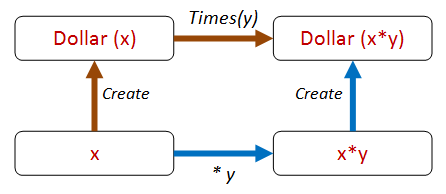Here’s that property expressed in code:

``````let ``create then times should be same as times then create`` start multiplier =
let d0 = Dollar.Create start
let d1 = d0.Times(multiplier)
let d2 = Dollar.Create (start * multiplier)
d1 = d2
``````

Great! Let’s see if it works!

``````Check.Quick ``create then times should be same as times then create``
// Falsifiable, after 1 test
``````

Oops – it doesn’t work!

Why not? Because we forgot that `Dollar` is a reference type and doesn’t compare equal by default!

As a result of this mistake, we have discovered a property that we might have overlooked! Let’s encode that before we forget.

``````let ``dollars with same amount must be equal`` amount =
let d1 = Dollar.Create amount
let d2 = Dollar.Create amount
d1 = d2

Check.Quick ``dollars with same amount must be equal``
// Falsifiable, after 1 test
``````

So now we need to fix this by adding support for `IEquatable` and so on.

You can do that if you like – I’m going to switch to F# record types and get equality for free!

### Dollar properties – version 3

Here’s the `Dollar` rewritten again:

``````type Dollar = {amount:int }
with
{amount = this.amount + add }
member this.Times multiplier  =
{amount = this.amount * multiplier }
static member Create amount  =
{amount=amount}
``````

And now our two properties are satisfied:

``````Check.Quick ``dollars with same amount must be equal``
// Ok, passed 100 tests.

Check.Quick ``create then times should be same as times then create``
// Ok, passed 100 tests.
``````

We can extend this approach for different paths. For example, we can extract the amount and compare it directly, like this: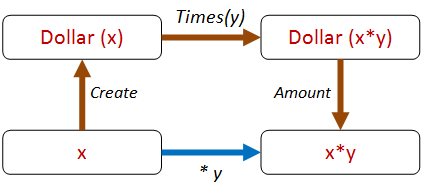The code looks like this:

``````let ``create then times then get should be same as times`` start multiplier =
let d0 = Dollar.Create start
let d1 = d0.Times(multiplier)
let a1 = d1.amount
let a2 = start * multiplier
a1 = a2

Check.Quick ``create then times then get should be same as times``
// Ok, passed 100 tests.
``````

And we can also include `Add` in the mix as well.

For example, we can do a `Times` followed by an `Add` via two different paths, like this: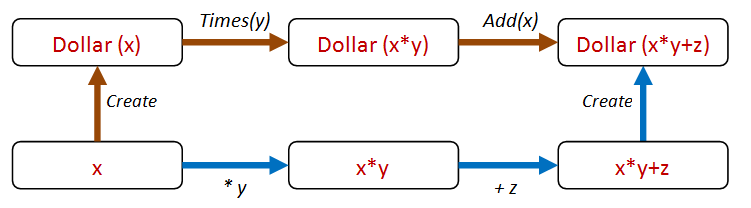And here’s the code:

``````let ``create then times then add should be same as times then add then create`` start multiplier adder =
let d0 = Dollar.Create start
let d1 = d0.Times(multiplier)
let directAmount = (start * multiplier) + adder
let d3 = Dollar.Create directAmount
d2 = d3

Check.Quick ``create then times then add should be same as times then add then create``
// Ok, passed 100 tests.
``````

So this “different paths, same result” approach is very fruitful, and we can generate lots of paths this way.

### Dollar properties – version 4

Shall we call it done then? I would say not!

We are beginning to get a whiff of a code smell. All this `(start * multiplier) + adder` code seems like a bit of duplicated logic, and could end up being brittle.

Can we abstract out some commonality that is present all these cases?

If we think about it, our logic is really just this:

• Transform the amount on the “inside” in some way.
• Transform the amount on the “outside” in the same way.
• Make sure that the results are the same.

But to test this, the `Dollar` class is going to have to support an arbitrary transform! Let’s call it `Map`!

Now all our tests can be reduced to this one property: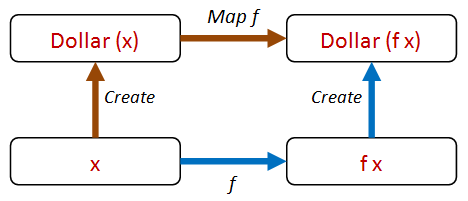Let’s add a `Map` method to `Dollar`. And we can also rewrite `Times` and `Add` in terms of `Map`:

``````type Dollar = {amount:int }
with
member this.Map f =
{amount = f this.amount}
member this.Times multiplier =
this.Map (fun a -> a * multiplier)
this.Map (fun a -> a + adder)
static member Create amount  =
{amount=amount}
``````

Now the code for our property looks like this:

``````let ``create then map should be same as map then create`` start f =
let d0 = Dollar.Create start
let d1 = d0.Map f
let d2 = Dollar.Create (f start)
d1 = d2
``````

But how can we test it now? What functions should we pass in?

Don’t worry! FsCheck has you covered! In cases like this, FsCheck will actually generate random functions for you too!

Try it – it just works!

``````Check.Quick ``create then map should be same as map then create``
// Ok, passed 100 tests.
``````

Our new “map” property is much more general than the original property using “times”, so we can eliminate the latter safely.

### Logging the function parameter

There’s a little problem with the property as it stands. If you want to see what the function is that FsCheck is generating, then Verbose mode is not helpful.

``````Check.Verbose ``create then map should be same as map then create``
``````

Gives the output:

``````0:
18
<fun:Invoke@3000>
1:
7
<fun:Invoke@3000>
-- etc
98:
47
<fun:Invoke@3000>
99:
36
<fun:Invoke@3000>
Ok, passed 100 tests.
``````

We can’t tell what the function values actually were.

However, you can tell FsCheck to show more useful information by wrapping your function in a special `F` case, like this:

``````let ``create then map should be same as map then create2`` start (F (_,f)) =
let d0 = Dollar.Create start
let d1 = d0.Map f
let d2 = Dollar.Create (f start)
d1 = d2
``````

And now when you use Verbose mode…

``````Check.Verbose ``create then map should be same as map then create2``
``````

… you get a detailed log of each function that was used:

``````0:
0
{ 0->1 }
1:
0
{ 0->0 }
2:
2
{ 2->-2 }
-- etc
98:
-5
{ -5->-52 }
99:
10
{ 10->28 }
Ok, passed 100 tests.
``````

Each `{ 2->-2 }`, `{ 10->28 }`, etc., represents the function that was used for that iteration.

## TDD vs. property-based testing

How does property-based testing (PBT) fit in with TDD? This is a common question, so let me quickly give you my take on it.

First off, TDD works with specific examples, while PBT works with universal properties.

As I said in the previous post, I think examples are useful as a way into a design, and can be a form of documentation. But in my opinion, relying only on example-based tests would be a mistake.

Property-based approaches have a number of advantages over example-based tests:

• Property-based tests are more general, and thus are less brittle.
• Property-based tests provide a better and more concise description of requirements than a bunch of examples.
• As a consequence, one property-based test can replace many, many, example-based tests.
• By generating random input, property-based tests often reveal issues that you have overlooked, such as dealing with nulls, missing data, divide by zero, negative numbers, etc.
• Property-based tests force you to think.
• Property-based tests force you to have a clean design.

These last two points are the most important for me. Programming is not a matter of writing lines of code, it is about creating a design that meets the requirements.

So, anything that helps you think deeply about the requirements and what can go wrong should be a key tool in your personal toolbox!

For example, in the Roman Numeral section, we saw that accepting `int` was a bad idea (the code broke!). We had a quick fix, but really we should model the concept of a `PositiveInteger` in our domain, and then change our code to use that type rather than just an `int`. This demonstrates how using PBT can actually improve your domain model, not just find bugs.

Similarly, introducing a `Map` method in the Dollar scenario not only made testing easier, but actually improved the usefulness of the Dollar “api”.

Stepping back to look at the big picture, though, TDD and property-based testing are not at all in conflict. They share the same goal of building correct programs, and both are really more about design than coding (think “Test-driven design” rather than “Test-driven development”).

## The end, at last

So that brings us to the end of another long post on property-based testing!

I hope that you now have some useful approaches that you can take away and apply to your own code base.

Next time, we’ll look at some real-world examples, and how you can create custom generators that match your domain.

The code samples used in this post are available on GitHub.